# TEXT Function

Convert numbers into text in a spreadsheet

## What is the Excel TEXT Function?

The Excel TEXT Function is used to convert numbers to text within a spreadsheet. Essentially, the function will convert a numeric value into a text string. TEXT is available in all versions of Excel.

### Formula

=Text(Value, format_text)

Where:

Value is the numerical value that we need to convert to text

Format_text is the format we want to apply

### When is the Excel TEXT Function required?

We use the TEXT function in the following circumstances:

1. When we want to display dates in a specified format
2. When we wish to display numbers in a specified format or in a more legible way
3. When we wish to combine numbers with text or characters

### Examples

#### 1. Basic example – Excel Text Function

With the following data, I need to convert the data to “d mmmm, yyyy” format. When we insert the text function, the result would look as follows: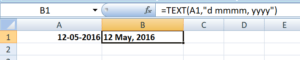#### 2. Using Excel TEXT with other functions

We use the old price and the discount given in cells A5 and B5. The quantity is given in C5. We wish to show some text along with the calculations. We wish to display the information as follows:

The final price is \$xxx

Where xxx would be the price in \$ terms.

For this, we can use the formula:

=”The final price is “&TEXT(A5*B5*C5, “\$###,###.00”)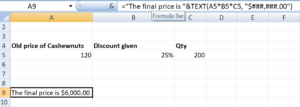The other way to do it by using the CONCATENATE function as shown below: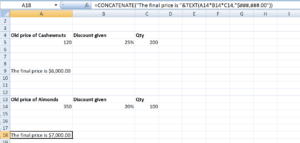#### 3. Combining the text given with data using TEXT function

When I use the date formula, I would get the result below: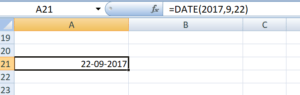Now, if we try to combine today’s date using CONCATENATE, Excel would give a weird result as shown below: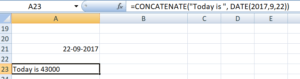What happened here was that dates that are stored as numbers by Excel were returned as numbers when the CONCATENATE function is used.

How to fix it?

To fix it, we need to use the Excel TEXT function. The formula to be used would be: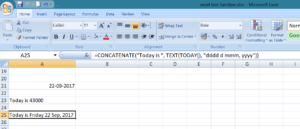#### 4. Adding zeros before numbers with variable lengths

We all know any zero’s added before numbers are automatically removed by Excel. However, if we need to keep those zeros then the TEXT function comes handy.  Let’s see an example to understand how to use this function.

We are given a 9-digit product code, but Excel removed the zeros before it. We can use TEXT as shown below and convert the product code into a 9-digit number: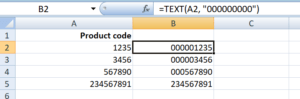In the above formula, we are given the format code containing 9-digit zeros, where the number of zeros is equal to the number of digits we wish to display.

#### 5. Converting telephone numbers to a specific format

If we wish to do the same for telephone numbers, it would involve the use of dashes and parentheses in format codes.

Here, I want to ensure the country code comes in brackets (). Hence the formula used is (##) ### ### ###. The # is the number of digits we wish to use.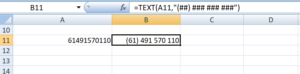### Format Code

It is quite easy to use TEXT Function in Excel but it works only when the correct format code is provided. Some frequently used format codes include:

CodeDescriptionExample
# (hash)It does not display extra zeros#.# displays a single decimal point. If we input 5.618, it will display 5.6.
0 (zero)It displays insignificant zeros#.000 would always display 3 decimals after the number. So if we input 5.68, it will display 5.680.
, (comma)It is a thousand separator###,### would put a thousands separator. So if we input 259890, it will display 259,890.

### If the Excel TEXT function isn’t working

Sometimes, the TEXT function will give an error “#NAME?”. This happens when we skip the quotation marks around the format code.

Let’s take an example to understand this.

If we input the formula =TEXT(A2, mm-dd-yy). It would give an error because the formula is incorrect and should be written this way: =TEXT(A2,”mm-dd-yy”).

### Tips

1. The data converted into text cannot be used for calculations. If needed, we should keep the original data in a hidden format and use it for other formulas.
2. The characters that can be included in the format code are:

 + Plus sign - Minus sign () Parenthesis : Colon {} Curly brackets = Equal sign ~ Tilde / Forward slash ! Exclamation mark <> Less than and greater than

1. TEXT function is language-specific. It requires the use of region-specific date and time format codes.

### Free Excel Course

Check out our Free Excel Crash Course and work your way toward becoming an expert financial analyst. Learn how to use Excel functions and create sophisticated financial analysis and financial modeling.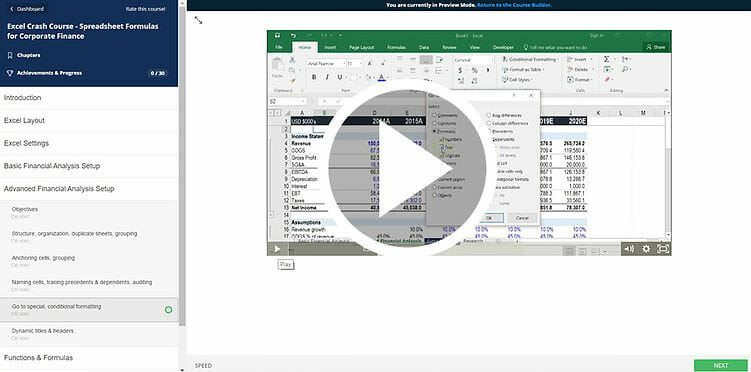Thanks for reading CFI’s guide to important Excel functions! By taking the time to learn and master these functions, you’ll significantly speed up your financial analysis. To learn more, check out these additional CFI resources:

• Excel Functions for Finance